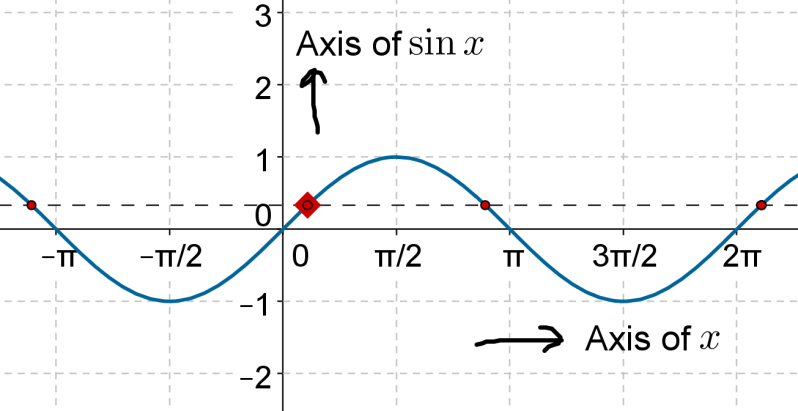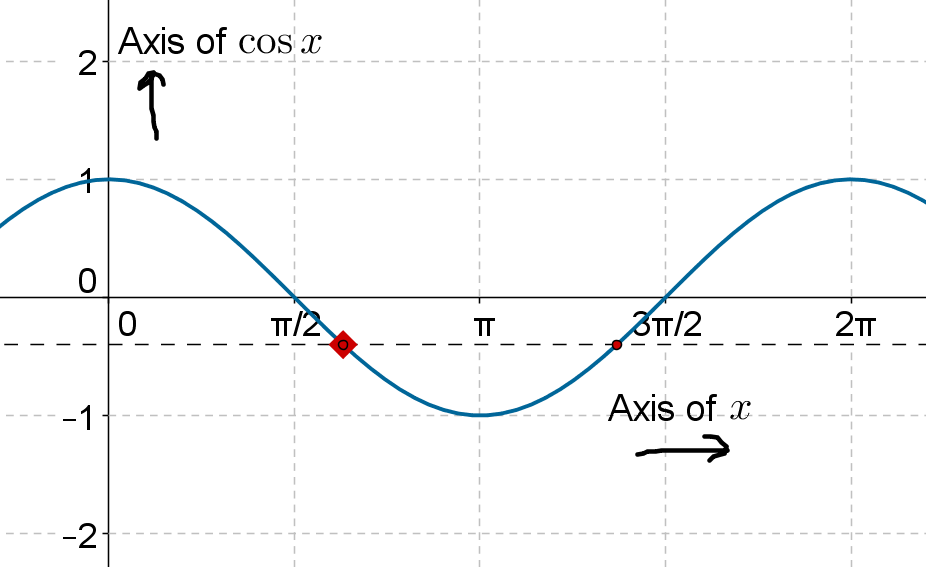# Inverse Trigonometric Ratios for Arbitrary Values

Inverse Trigonometric Ratios for Arbitrary Values

## Introduction:

Suppose that $$\sin \theta = \frac{1}{2}$$. It is easy to invert this and write,

$\theta = {\sin ^{ - 1}}\left( {\frac{1}{2}} \right) = \frac{\pi }{6}$

Similarly, $$\cos \theta = \frac{1}{2}$$ can be inverted to write,

$\theta = {\cos ^{ - 1}}\left( {\frac{1}{2}} \right) = \frac{\pi }{3}$

Now, suppose that we have the equation $$\sin \theta = \frac{3}{5}$$. How do we invert this equation and write the value of $$\theta$$? Since there is no readily available value of $$\theta$$ (in our minds) for which $$\sin \theta = \frac{3}{5}$$, we do the inversion by simply writing,

$\theta = {\sin ^{ - 1}}\left( {\frac{3}{5}} \right)$

We do not then write a specific value for $$\theta$$ (unless asked for explicitly – in which case you will require trigonometric tables to locate the angle for which $$\sin \theta = \frac{3}{5}$$).

Note: The actual value of $${\sin ^{ - 1}}\left( {\frac{3}{5}} \right)$$ is about 36.90. You can check this using calculator:

$\sin \left( {{{36.9}^0}} \right) \approx 0.60$

As another example, consider the equation $$\cos \theta = \frac{1}{4}$$. Inverting this, we can write:

$\theta = {\cos ^{ - 1}}\left( {\frac{1}{4}} \right)$

Note: We can’t do much more than this without a calculator or trigonometric tables, since we can’t mentally calculate the angle whose cosine will be $$\frac{1}{4}$$. However, a calculator will tell you that,

${\cos ^{ - 1}}\left( {\frac{1}{4}} \right) \approx {75.5^0}$

## Solved Examples:

Example 1: Find all values of $$x$$ such that $$\sin x = \frac{1}{3}$$.

Solution: In the principal range of the $${\sin ^{ - 1}}$$ operation, the correct value of $$x$$ will be

$x = {\sin ^{ - 1}}\left( {\frac{1}{3}} \right)$

Observe the following figure, which shows the intersection of $$y = \frac{1}{3}$$ with $$y = \sin x$$:The highlighted point corresponds to the value of $$x$$ in the principal range, which we can write as $${\sin ^{ - 1}}\left( {\frac{1}{3}} \right)$$ . Another value of $$x$$ which we can observe from this figure is

$x = \pi - {\sin ^{ - 1}}\left( {\frac{1}{3}} \right)$

In the interval $$\left[ {0,2\pi } \right]$$, these are the only two values of $$x$$ for which $$\sin x = \frac{1}{3}$$. Now, we can generalize and write other values of $$x$$ for which $$\sin x = \frac{1}{3}$$ ($$n$$ lies in the set of integers):

$\boxed{x = 2n\pi + {\sin ^{ - 1}}\left( {\frac{1}{3}} \right),2n\pi + \pi - {\sin ^{ - 1}}\left( {\frac{1}{3}} \right)}$

Example 2: Find all values of $$x$$ such that $$\cos x = - \frac{2}{5}$$.

Solution:The principal range of the $${\cos ^{ - 1}}$$ operation is the interval $$\left[ {0,\pi } \right]$$. The following figure shows the intersection of $$y = - \frac{2}{5}$$ with $$y = \cos x$$:In $$\left[ {0,\pi } \right]$$, we see one intersection point. We can write the $$x$$-coordinate of this intersection point as

${x_p} = {\cos ^{ - 1}}\left( { - \frac{2}{5}} \right)$

A calculator will tell you that this value is roughly around 113.60.

We can also see another possible solution in the interval $$\left[ {0,2\pi } \right]$$. Recall that $$\cos x = \cos \left( {2\pi - x} \right)$$. Thus, this second solution is

$2\pi - {x_p} = 2\pi - {\cos ^{ - 1}}\left( { - \frac{2}{5}} \right)$

Now, we can write the general set of solutions as follows ($$n$$ lies in the set of integers):

$\boxed{x = 2n\pi + {\cos ^{ - 1}}\left( { - \frac{2}{5}} \right),2n\pi + 2\pi - {\cos ^{ - 1}}\left( { - \frac{2}{5}} \right)}$Challenge: Find all values of $$x$$ such that $$\sin x = - \frac{1}{4}$$

Tip: Use a similar approach as in example-1.

Trigonometry